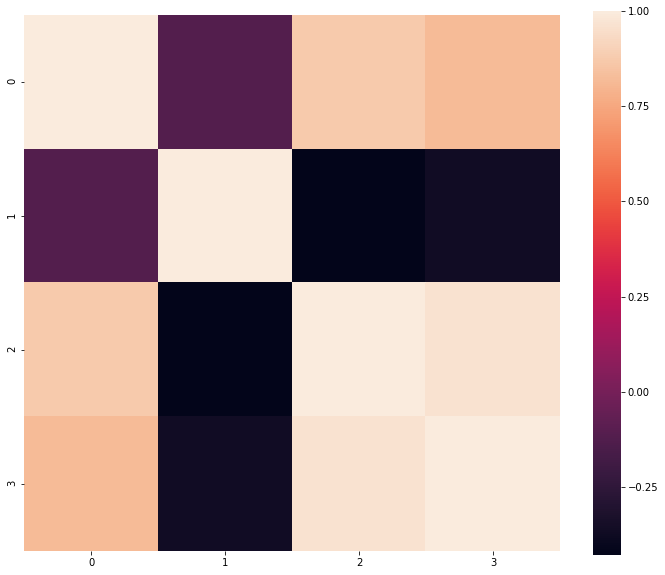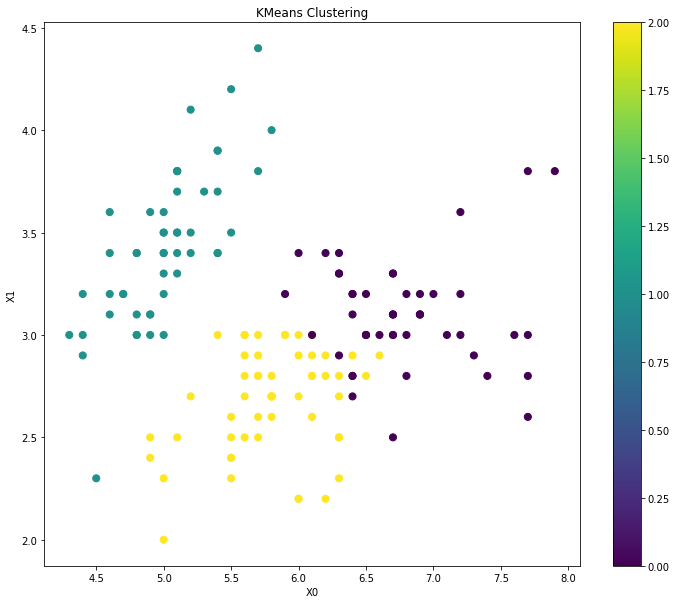How to do KMeans Clustering in Python?
MACHINE LEARNING RECIPES DATA CLEANING PYTHON DATA MUNGING PANDAS CHEATSHEET     ALL TAGS

# How to do KMeans Clustering in Python?

This recipe helps you do KMeans Clustering in Python

0
In :
```## How to do KMeans Clustering in Python
def Snippet_157():
print()
print(format('How to do KMeans Clustering in Python','*^82'))

import warnings
warnings.filterwarnings("ignore")

from sklearn import datasets
from sklearn.preprocessing import StandardScaler
from sklearn.cluster import KMeans
import pandas as pd
import seaborn as sns
import matplotlib.pyplot as plt

X = iris.data; data = pd.DataFrame(X)
cor = data.corr()
# Plot correlation using seaborn
fig = plt.figure(figsize=(12,10));
sns.heatmap(cor, square = True); plt.show()

# Standarize features
scaler = StandardScaler()
X_std = scaler.fit_transform(X)

# Conduct KMeans Clustering
clt = KMeans(n_clusters=3)

# Train model
model = clt.fit(X_std)

# Predict clusters
clusters = pd.DataFrame(model.fit_predict(X_std))
data['Cluster'] = clusters

# Visualise cluster membership
fig = plt.figure(figsize=(12,10)); ax = fig.add_subplot(111)
scatter = ax.scatter(data,data, c=data['Cluster'],s=50)
ax.set_title('KMeans Clustering')
ax.set_xlabel('X0'); ax.set_ylabel('X1')
plt.colorbar(scatter); plt.show()

Snippet_157()
```
```**********************How to do KMeans Clustering in Python***********************
```#### Relevant Projects

##### Data Science Project - Instacart Market Basket Analysis
Data Science Project - Build a recommendation engine which will predict the products to be purchased by an Instacart consumer again.

##### Resume parsing with Machine learning - NLP with Python OCR and Spacy
In this machine learning resume parser example we use the popular Spacy NLP python library for OCR and text classification.

##### PySpark Tutorial - Learn to use Apache Spark with Python
PySpark Project-Get a handle on using Python with Spark through this hands-on data processing spark python tutorial.

##### Music Recommendation System Project using Python and R
Machine Learning Project - Work with KKBOX's Music Recommendation System dataset to build the best music recommendation engine.

##### Sequence Classification with LSTM RNN in Python with Keras
In this project, we are going to work on Sequence to Sequence Prediction using IMDB Movie Review Dataset​ using Keras in Python.

##### Predict Churn for a Telecom company using Logistic Regression
Machine Learning Project in R- Predict the customer churn of telecom sector and find out the key drivers that lead to churn. Learn how the logistic regression model using R can be used to identify the customer churn in telecom dataset.

##### Choosing the right Time Series Forecasting Methods
There are different time series forecasting methods to forecast stock price, demand etc. In this machine learning project, you will learn to determine which forecasting method to be used when and how to apply with time series forecasting example.

##### Deep Learning with Keras in R to Predict Customer Churn
In this deep learning project, we will predict customer churn using Artificial Neural Networks and learn how to model an ANN in R with the keras deep learning package.

##### Machine Learning or Predictive Models in IoT - Energy Prediction Use Case
In this machine learning and IoT project, we are going to test out the experimental data using various predictive models and train the models and break the energy usage.

##### Predict Macro Economic Trends using Kaggle Financial Dataset
In this machine learning project, you will uncover the predictive value in an uncertain world by using various artificial intelligence, machine learning, advanced regression and feature transformation techniques.